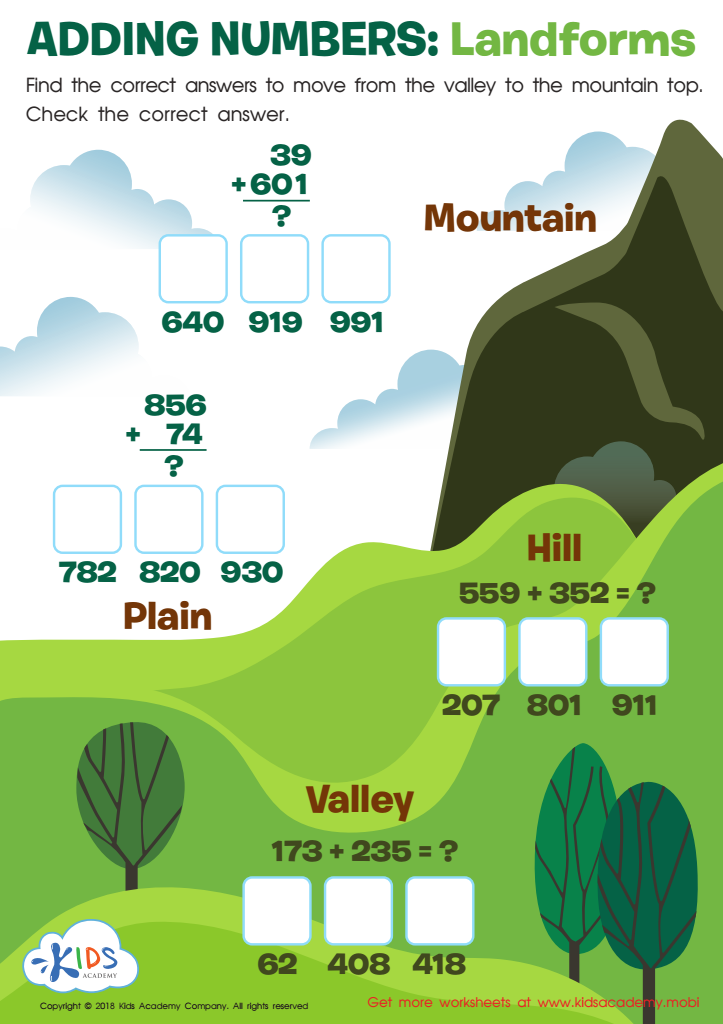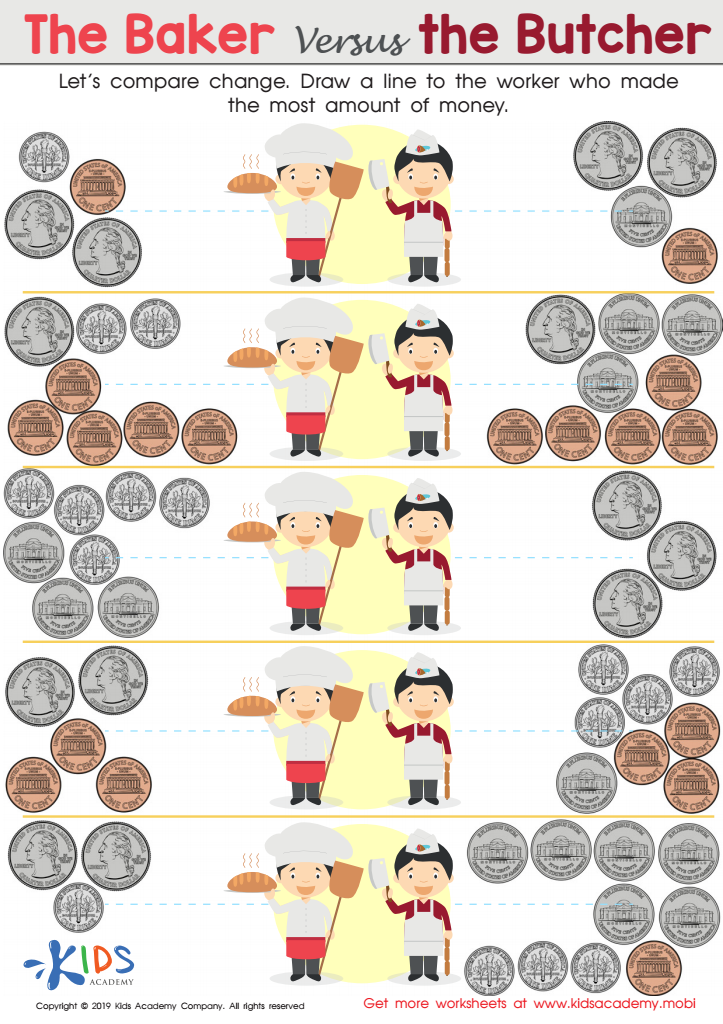21 filtered results

Clear all filters
Favorites
Online only

21 filtered results

Difficulty Level

Standards

Introducing our Easy Addition & Subtraction worksheets, specially designed for Grade 2 students! With these engaging worksheets, your young learners can effortlessly grasp the fundamentals of addition and subtraction. Each worksheet presents a variety of colorful and fun exercises, allowing children to enhance their numerical skills while having a blast. The accompanying answer key ensures easy evaluation, helping both students and teachers track progress effectively. Our Easy Addition & Subtraction worksheets with answers for Grade 2 provide an excellent resource for building a strong foundation in math, fostering confidence and success in every child's mathematical journey.

Favorites
Online only
• Easy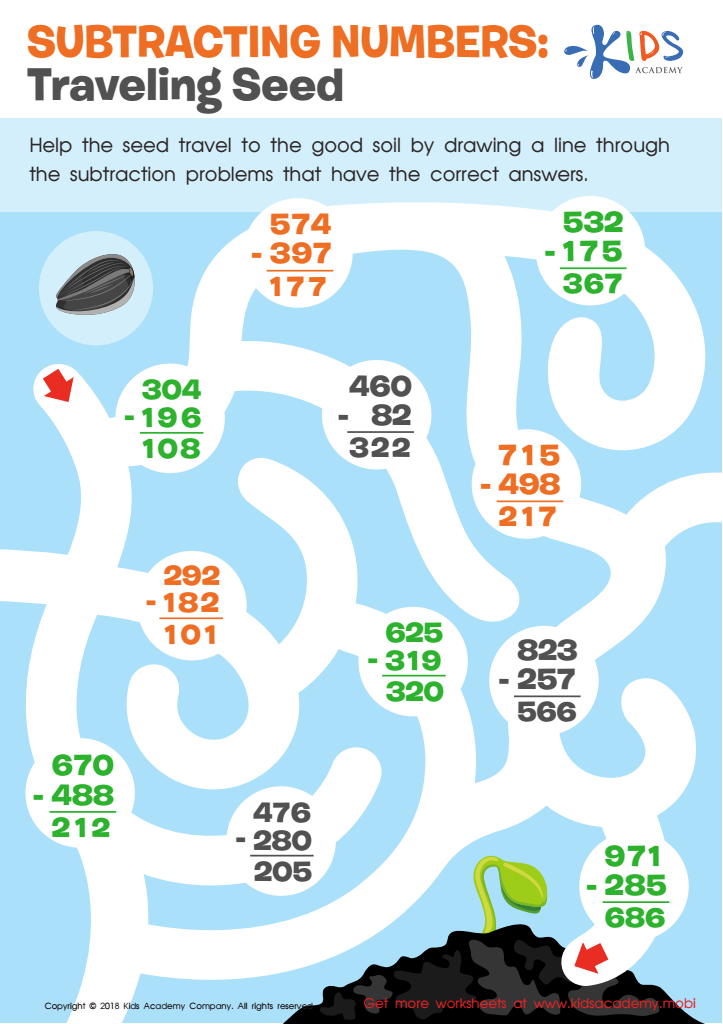Subtracting Numbers: Traveling Seeds Worksheet
Introduce students to subtracting equations with this math worksheet! Engaging images will help them practice. Draw a line through the subtraction problems with the right answers to help the image reach the good soil. Let your students solve the math maze and have fun while they learn!
Subtracting Numbers: Traveling Seeds Worksheet
Worksheet
Subtracting Numbers: Traveling Seeds Worksheet
If you have introduced your students to subtracting equations, then you will need this simple …
Worksheet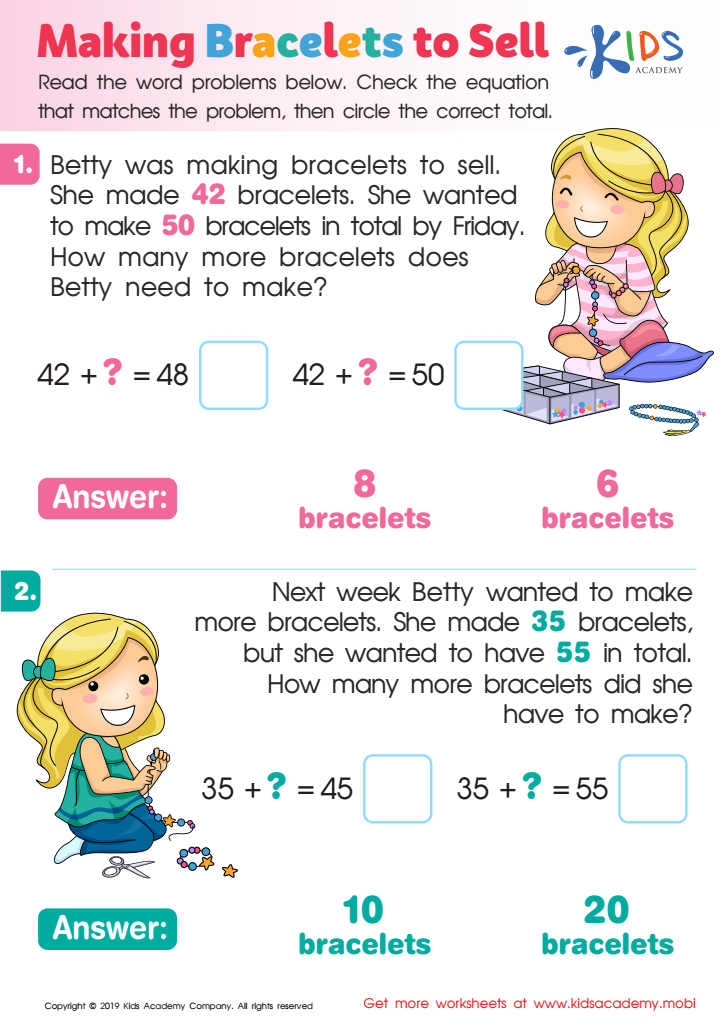Making Bracelets to Sell Worksheet
Got kids who love crafting? This worksheet will be right up their alley! Let them use their creative and problem-solving skills to check equations and decide the correct total. It's just like making beads into a bracelet but on paper! Print out this downloadable PDF and get ready for a fun craft session.
Making Bracelets to Sell Worksheet
Worksheet
Making Bracelets to Sell Worksheet
Do your kids love making crafts? If they do, then they would love this colorful …
Worksheet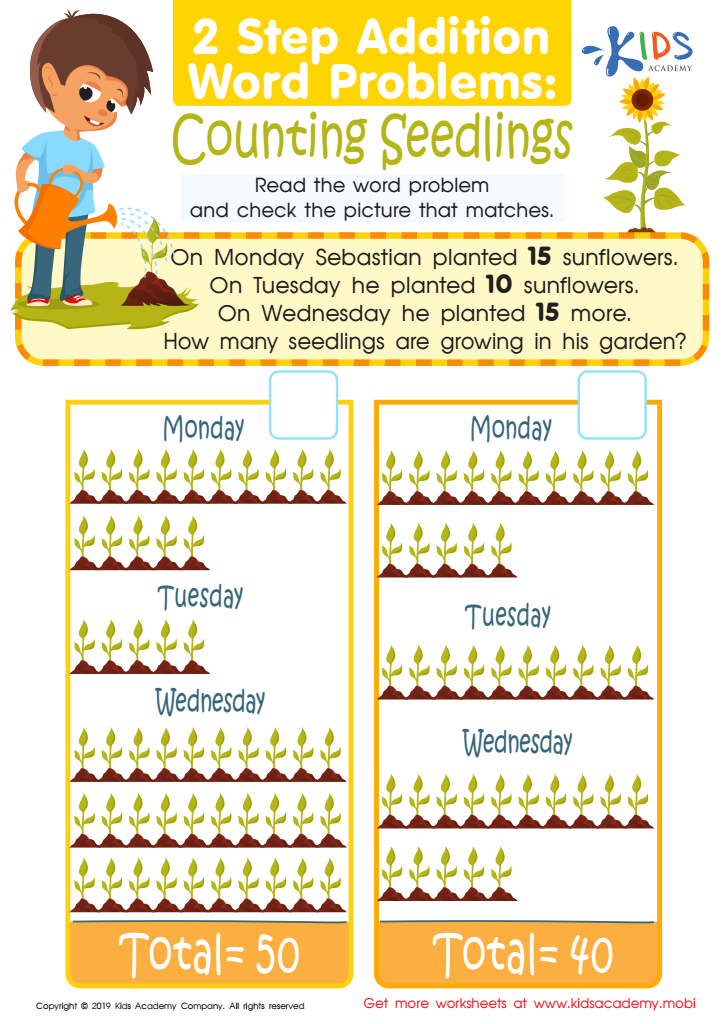Counting Seedlings Worksheet
Understanding math word problems is key. Multiple steps can prove challenging - this free worksheet provides one-to-one picture representation to help kids solve multi-step addition word problems. Strengthen addition skills by choosing the matching picture to the answer.
Counting Seedlings Worksheet
Worksheet
Counting Seedlings Worksheet
When it comes to math word problems, understanding what is being asked is a key. …
Worksheet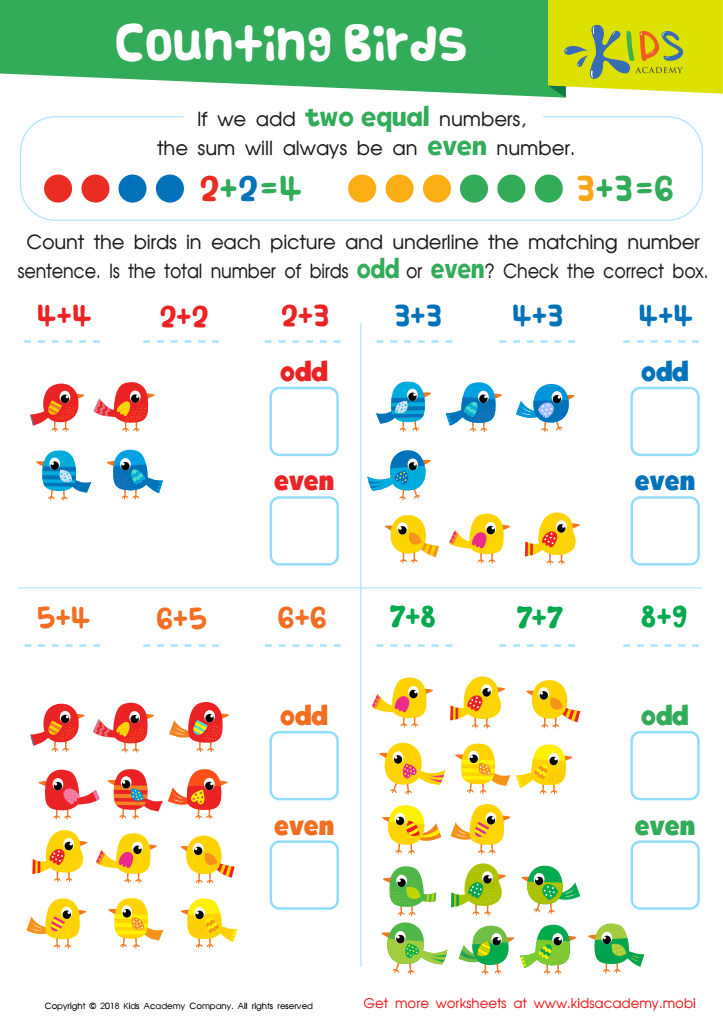Counting Birds Worksheet
Help your child count and underline the matching number sentence in the worksheet. Ask them if the total number of birds in each picture is odd or even, then check the correct box. When two equal numbers are added, the sum is always an even number (e.g. 2+2=4, 4+4=8, both divisible by 2).
Counting Birds Worksheet
Worksheet
Counting Birds Worksheet
When two equal numbers are added, the sum of the two numbers will always be …